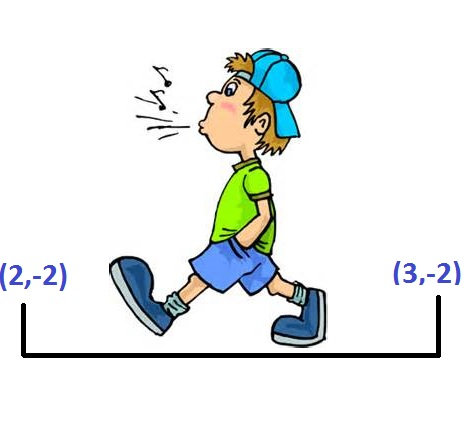# Walk the grid

Probability Level 5You start at the point $(x,y) = (1,1)$ in a Cartesian coordinate system.

Every second you move randomly 1 unit either up, down, left or right.

What is the expected value for the number of seconds it will take for you to land on a point $(5m, 5n)$ where $m$ and $n$ are integers?

Clarification: You can walk anywhere in all four quadrants of the Cartesian coordinate system. $m$ and/or $n$ can be negative.

×